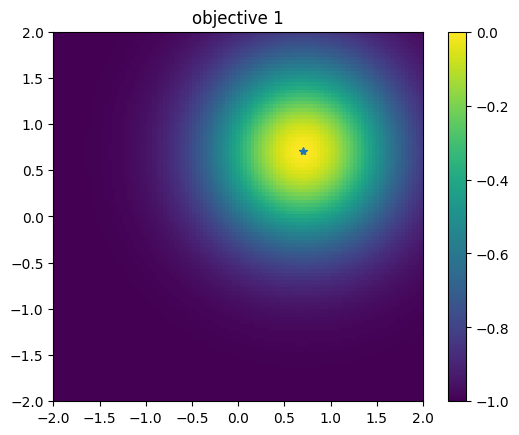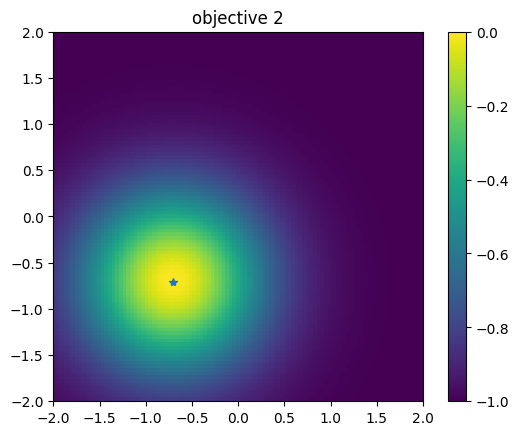# Multi-objective optimization

When there are multiple objective functions ($$p$$) to be optimized, multi-objective optimization is used.
Note that in this tutorial, “solution” means the pair of objective functions $$y = (y_1(x), y_2(x), \dots, y_p(x))$$.
Define the solution size relation $$\prec$$ as follows.

$$y \prec y^{'}\Longleftrightarrow \forall \ i \le p, y_i \le y^{'}_i \land \exists \ j \le p, y_j < y^{'}_j$$

A Pareto solution (in the maximization problem) is one in which no solution is larger than itself satisfying the above relations.
In other words, if you try to improve the value of any objective function, one of the other objective functions will deteriorate.
When there is a trade-off between objective functions, there are multiple Pareto solutions, and the numerical task is to find them efficiently.

PHYSBO implements a Bayesian optimization method to find Pareto solutions efficiently.

:

import numpy as np
import matplotlib.pyplot as plt
import physbo
%matplotlib inline


## Test functions

In this tutorial, we will use VLMOP2, which is a benchmark function for multi-objective optimization.
The number of objective functions is two.
$\begin{split}\begin{split} y_1(\vec{x}) &= 1 - \exp\left[-\sum_{i=1}^N\left( x_i - 1/\sqrt{N}\right)^2\right] \\ y_2(\vec{x}) &= 1 - \exp\left[-\sum_{i=1}^N\left( x_i + 1/\sqrt{N}\right)^2\right], \end{split}\end{split}$

where $$y_1$$ and $$y_2$$ have minimums at $$x_1 = x_2 = \cdots x_N = 1/\sqrt{N}$$ and $$x_1 = x_2 = \cdots x_N = -1/\sqrt{N}$$, respectively, both of which are 0. Also, the upper bound is 1.

Since PHYSBO solves a maximization problem, the objective function is again multiplied by -1.

• Refernce

• Van Veldhuizen, David A. Multiobjective evolutionary algorithms: classifications, analyses, and new innovations. No. AFIT/DS/ENG/99-01. AIR FORCE INST OF TECH WRIGHT-PATTERSONAFB OH SCHOOL OF ENGINEERING, 1999.

:

def vlmop2_minus(x):
n = x.shape
y1 = 1 - np.exp(-1 * np.sum((x - 1/np.sqrt(n)) ** 2, axis = 1))
y2 = 1 - np.exp(-1 * np.sum((x + 1/np.sqrt(n)) ** 2, axis = 1))

return np.c_[-y1, -y2]


## Preparation of search candidate data

Let the input space $$\vec{x}$$ be two-dimensional, and generate a grid of candidate points on [-2, 2] × [-2, 2].

:

import itertools
a = np.linspace(-2,2,101)
test_X = np.array(list(itertools.product(a, a)))

:

test_X

:

array([[-2.  , -2.  ],
[-2.  , -1.96],
[-2.  , -1.92],
...,
[ 2.  ,  1.92],
[ 2.  ,  1.96],
[ 2.  ,  2.  ]])

:

test_X.shape

:

(10201, 2)


## Definition of simulator

:

class simulator(object):
def __init__(self, X):
self.t = vlmop2_minus(X)

def __call__( self, action):
return self.t[action]

:

simu = simulator(test_X)


## Plotting the functions

Let’s plot each of the two objective functions. The first objective function has a peak in the upper right corner, and the second objective function has a trade-off with a peak in the lower left corner (The star is the position of the peak.).

### First objective function

:

plt.figure()
plt.imshow(simu.t[:,0].reshape((101,101)), vmin=-1.0, vmax=0.0, origin="lower", extent=[-2.0, 2.0, -2.0, 2.0])
plt.title("objective 1")
plt.colorbar()
plt.plot([1.0/np.sqrt(2.0)], [1.0/np.sqrt(2.0)], '*')
plt.show()### Second objective function

:

# plot objective 2
plt.figure()
plt.imshow(simu.t[:,1].reshape((101,101)), vmin=-1.0, vmax=0.0, origin="lower", extent=[-2.0, 2.0, -2.0, 2.0])
plt.title("objective 2")
plt.colorbar()
plt.plot([-1.0/np.sqrt(2.0)], [-1.0/np.sqrt(2.0)], '*')
plt.show()## Setting policy

Use physbo.search.discrete_multi.policy for multi-objective optimization.
Specify the number of objective functions in num_objectives.
:

policy = physbo.search.discrete_multi.policy(test_X=test_X, num_objectives=2)
policy.set_seed(0)


As with the usual usage of physbo.search.discrete.policy (with one objective function), optimization is done by calling the random_search or bayes_search methods. The basic API and usage are roughly the same as discrete.policy.

### Checking results

#### History of evaluation values

[ ]:

res_random.fx[0:res_random.num_runs]


#### Obtaining the Pareto solution

:

front, front_num = res_random.export_pareto_front()
front, front_num

:

(array([[-0.95713719, -0.09067194],
[-0.92633083, -0.29208351],
[-0.63329589, -0.63329589],
[-0.52191048, -0.72845916],
[-0.26132949, -0.87913689],
[-0.17190645, -0.91382463]]),
array([40,  3, 19, 16, 29, 41]))


#### Plotting the solution (evaluated value)

Note again that the space to be plotted is $$y = (y_1, y_2)$$ and not $$x = (x_1, x_2)$$.

The red plot is the Pareto solution.

:

def plot_pareto_front(res):
front, front_num = res.export_pareto_front()
dominated = [i for i in range(res.num_runs) if i not in front_num]
points = res.fx[dominated, :]

plt.figure(figsize=(7, 7))
plt.scatter(res.fx[dominated,0], res.fx[dominated,1], c = "blue")
plt.scatter(front[:, 0], front[:, 1], c = "red")
plt.title('Pareto front')
plt.xlabel('Objective 1')
plt.ylabel('Objective 2')
plt.xlim([-1.0,0.0])
plt.ylim([-1.0,0.0])

:

plot_pareto_front(res_random)#### Calculate the volume of the dominated region

A solution that is not a Pareto solution, i.e., a solution $$y$$ for which there exists a solution $$y'$$ that is better than itself, is called a inferior solution ($$\exists y' y\prec y'$$). The volume of the inferior solution region, which is the space occupied by inferior solutions in the solution space (a subspace of the solution space), is one of the indicators of the results of multi-objective optimization. The larger this value is, the more good Pareto solutions are obtained.res_random.pareto.volume_in_dominance(ref_min, ref_max) calculates the volume of the inferior solution region in the hyper-rectangle specified by ref_min and ref_max.

:

res_random.pareto.volume_in_dominance([-1,-1],[0,0])

:

0.2376881844865093


## Bayesian optimization

For bayes_search in the multi-objective case, score can be selected from the following method

• HVPI (HyperVolume-based Probability of Improvement)

• EHVI (Expected Hyper-Volume Improvement)

• TS (Thompson Sampling)

The following 50 evaluations (10 random searches + 40 Bayesian optimizations) will be performed with different scores.

### HVPI (HyperVolume-based Probability of Improvement)

The improvement probability of a non-dominated region in a multi-dimensional objective function space is obtained as a score.

• Reference

• Couckuyt, Ivo, Dirk Deschrijver, and Tom Dhaene. “Fast calculation of multiobjective probability of improvement and expected improvement criteria for Pareto optimization.” Journal of Global Optimization 60.3 (2014): 575-594.

[ ]:

policy = physbo.search.discrete_multi.policy(test_X=test_X, num_objectives=2)
policy.set_seed(0)

policy.random_search(max_num_probes=10, simulator=simu)
res_HVPI = policy.bayes_search(max_num_probes=40, simulator=simu, score='HVPI', interval=10)


#### Plotting the Pareto solution

We can see that more Pareto solutions are obtained compared to random sampling.

:

plot_pareto_front(res_HVPI)#### Volume of dominated region

:

res_HVPI.pareto.volume_in_dominance([-1,-1],[0,0])

:

0.32877907991633726


### EHVI (Expected Hyper-Volume Improvement)

The expected improvement of the non-dominated region in the multi-dimensional objective function space is obtained as score.

• Reference

• Couckuyt, Ivo, Dirk Deschrijver, and Tom Dhaene. “Fast calculation of multiobjective probability of improvement and expected improvement criteria for Pareto optimization.” Journal of Global Optimization 60.3 (2014): 575-594.

[ ]:

policy = physbo.search.discrete_multi.policy(test_X=test_X, num_objectives=2)
policy.set_seed(0)

policy.random_search(max_num_probes=10, simulator=simu)
res_EHVI = policy.bayes_search(max_num_probes=40, simulator=simu, score='EHVI', interval=10)


#### Plotting the Pareto solution

:

plot_pareto_front(res_EHVI)#### Volume of dominated region

:

res_EHVI.pareto.volume_in_dominance([-1,-1],[0,0])

:

0.3200467412741881


### TS (Thompson Sampling)

In Thompson Sampling for the single objective case, at each candidate (test_X), sampling is performed from the posterior distribution of the objective function, and the candidate with the largest value is recommended as the next search point. In the multi-objective case, one candidate is randomly selected as the next search point from among the candidates with the maximum value based on the Pareto rule for the sampled values, i.e., the Pareto-optimal candidates.

• Reference

• Yahyaa, Saba Q., and Bernard Manderick. “Thompson sampling for multi-objective multi-armed bandits problem.” Proc. Eur. Symp. Artif. Neural Netw., Comput. Intell. Mach. Learn.. 2015.

[ ]:

policy = physbo.search.discrete_multi.policy(test_X=test_X, num_objectives=2)
policy.set_seed(0)

policy.random_search(max_num_probes=10, simulator=simu)
res_TS = policy.bayes_search(max_num_probes=40, simulator=simu, score='TS', interval=10, num_rand_basis=5000)


#### Plotting the Pareto solution

:

plot_pareto_front(res_TS)#### Volume of dominated region

:

res_TS.pareto.volume_in_dominance([-1,-1],[0,0])

:

0.16415446221006114